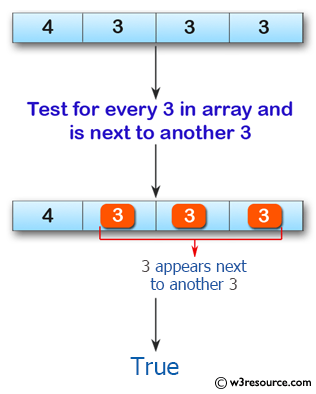﻿ Swift Array Programming Exercise: Test if every 3 that appears in a given array of integers is next to another 3 - w3resource# Swift Array Programming Exercises: Test if every 3 that appears in a given array of integers is next to another 3

## Swift Array Programming: Exercise-36 with Solution

Write a Swift program to test if every 3 that appears in a given array of integers is next to another 3.

Pictorial Presentation:Sample Solution:

Swift Code:

``````func three_two(array_nums: [Int]) -> Bool {
var x = 0
while x < array_nums.count-1 {
if array_nums[x] == 3 && array_nums[x+1] != 3
{
return false
}
else if array_nums[x] == 3 && array_nums[x+1] == 3
{
x += 1
}
x += 1
}

if array_nums[array_nums.count-1] == 2
{
return false
}

return true
}

print(three_two(array_nums: [4, 3, 3, 3]))
print(three_two(array_nums: [3, 1, 4]))
print(three_two(array_nums: [3, 3, 4, 3]))
```
```

Sample Output:

```true
false
true
```

Swift Programming Code Editor:

Improve this sample solution and post your code through Disqus

What is the difficulty level of this exercise?

﻿